# Matrix factorization method

(diff) ← Older revision | Latest revision (diff) | Newer revision → (diff)

matrix forward-backward substitution method

A method for solving finite-difference systems that approximate boundary value problems for systems of ordinary differential equations in one-dimensional problems, and for elliptic equations in two-dimensional problems.

The solution of the three-point difference schemewhere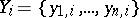is an unknown grid vector,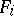is the right-hand side vector andare given square matrices, under the boundary conditionsis sought for, as in the scalar case, in the form(*)

The coefficients (the matrixand the vector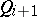) are determined from the recurrence relations ( "forward substitution" )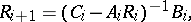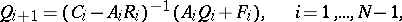while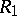andare given by the left boundary condition:Theare calculated by formula (*) ( "backward substitution" ), and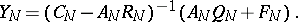There is stability of this method to rounding errors under the conditions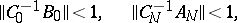which implies that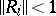,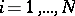(see ). A different form of the stability conditions is also available (see , ). The matrix factorization method is applied also to two-point difference schemes (see ). A variant in which inversion of matrices is replaced by orthogonalization is also used (see ).

How to Cite This Entry:
Matrix factorization method. Encyclopedia of Mathematics. URL: http://encyclopediaofmath.org/index.php?title=Matrix_factorization_method&oldid=15766
This article was adapted from an original article by T.A. Germogenova (originator), which appeared in Encyclopedia of Mathematics - ISBN 1402006098. See original article